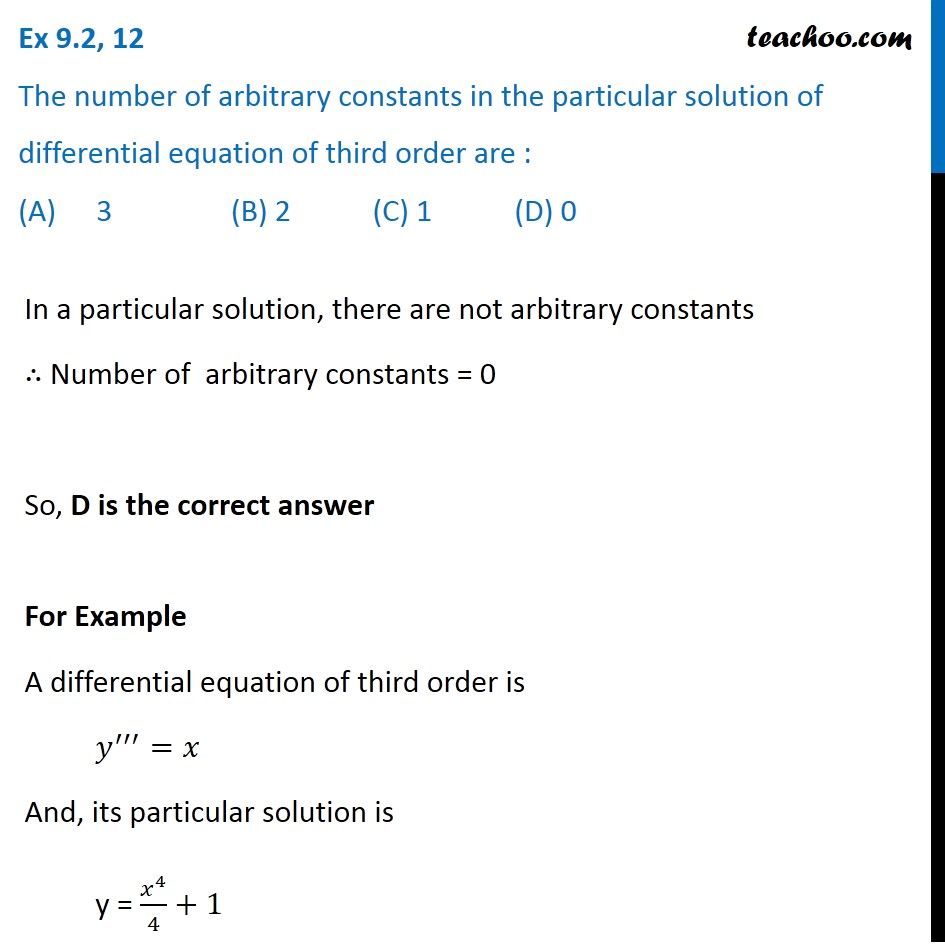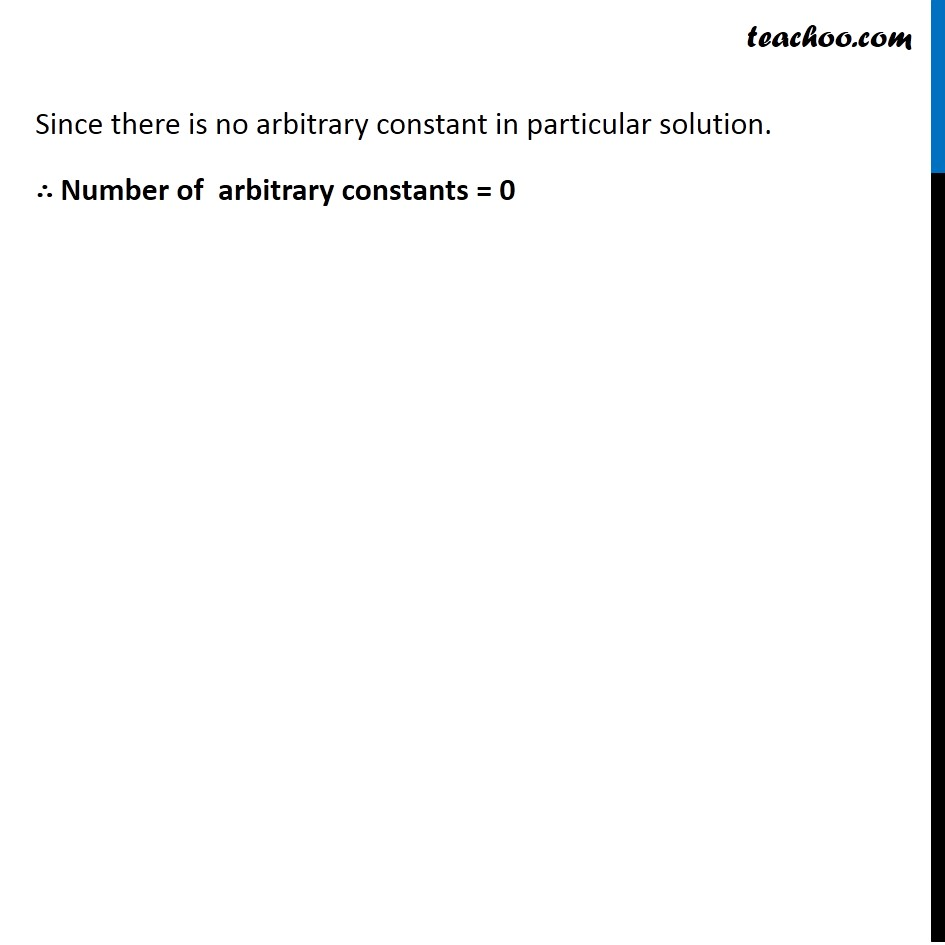Ex 9.2

Chapter 9 Class 12 Differential Equations
Serial order wiseLearn in your speed, with individual attention - Teachoo Maths 1-on-1 Class

### Transcript

Ex 9.2, 12 The number of arbitrary constants in the particular solution of differential equation of third order are : (A) 3 (B) 2 (C) 1 (D) 0 In a particular solution, there are not arbitrary constants ∴ Number of arbitrary constants = 0 So, D is the correct answer For Example A differential equation of third order is 𝑦^′′′=𝑥 And, its particular solution is y = 𝑥^4/4+1 Since there is no arbitrary constant in particular solution. ∴ Number of arbitrary constants = 0